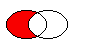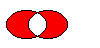# QRegion¶

The `QRegion` class specifies a clip region for a painter. More## Detailed Description¶

`QRegion` is used with `setClipRegion()` to limit the paint area to what needs to be painted. There is also a `repaint()` function that takes a `QRegion` parameter. `QRegion` is the best tool for minimizing the amount of screen area to be updated by a repaint.

This class is not suitable for constructing shapes for rendering, especially as outlines. Use `QPainterPath` to create paths and shapes for use with `QPainter` .

`QRegion` is an implicitly shared class.

### Creating and Using Regions¶

A region can be created from a rectangle, an ellipse, a polygon or a bitmap. Complex regions may be created by combining simple regions using `united()` , `intersected()` , `subtracted()` , or `xored()` (exclusive or). You can move a region using `translate()` .

You can test whether a region `isEmpty()` or if it `contains()` a `QPoint` or `QRect` . The bounding rectangle can be found with `boundingRect()` .

Iteration over the region (with `begin()` , `end()` , or C++11 ranged-for loops) gives a decomposition of the region into rectangles.

Example of using complex regions:

```class MyWidget (QWidget):
# ...
def paintEvent(self):
r1 = QRegion(QRect(100, 100, 200, 80), QRegion.Ellipse) # r1: elliptic region
)
r2 = QRect(100, 120, 90, 30)   # r2: rectangular region
r3 = r1.intersected(r2)        # r3: intersection

painter = QPainter(self)
painter.setClipRegion(r3)
...                            # paint clipped graphics
```
class `QRegion`

QRegion(bitmap)

QRegion(pa[, fillRule=Qt.OddEvenFill])

QRegion(r[, t=Rectangle])

QRegion(region)

QRegion(x, y, w, h[, t=Rectangle])

param w

`int`

param x

`int`

param y

`int`

param h

`int`

param fillRule

`FillRule`

param bitmap

`QBitmap`

param region

`QRegion`

param r

`QRect`

param t

`RegionType`

param pa

`QPolygon`

Constructs an empty region.

Constructs a rectangular or elliptic region.

If `t` is `Rectangle` , the region is the filled rectangle (`x` , `y` , `w` , `h` ). If `t` is `Ellipse` , the region is the filled ellipse with center at (`x` + `w` / 2, `y` + `h` / 2) and size (`w` ,``h`` ).

`PySide2.QtGui.QRegion.``RegionType`

Specifies the shape of the region to be created.

Constant

Description

QRegion.Rectangle

the region covers the entire rectangle.

QRegion.Ellipse

the region is an ellipse inside the rectangle.

`PySide2.QtGui.QRegion.``begin`()
Return type

`QRect`

Returns a `const_iterator` pointing to the beginning of the range of non-overlapping rectangles that make up the region.

The union of all the rectangles is equal to the original region.

`rbegin()` `cbegin()` `end()`

`PySide2.QtGui.QRegion.``boundingRect`()
Return type

`QRect`

Returns the bounding rectangle of this region. An empty region gives a rectangle that is `isNull()` .

`PySide2.QtGui.QRegion.``cbegin`()
Return type

`QRect`

Same as `begin()` .

`PySide2.QtGui.QRegion.``cend`()
Return type

`QRect`

Same as `end()` .

`PySide2.QtGui.QRegion.``contains`(p)
Parameters

p`QPoint`

Return type

`bool`

`PySide2.QtGui.QRegion.``contains`(r)
Parameters

r`QRect`

Return type

`bool`

`PySide2.QtGui.QRegion.``end`()
Return type

`QRect`

Returns a `const_iterator` pointing to one past the end of non-overlapping rectangles that make up the region.

The union of all the rectangles is equal to the original region.

`rend()` `cend()` `begin()`

`PySide2.QtGui.QRegion.``intersected`(r)
Parameters

r`QRect`

Return type

`QRegion`

`PySide2.QtGui.QRegion.``intersected`(r)
Parameters
Return type

`QRegion`

`PySide2.QtGui.QRegion.``intersects`(r)
Parameters

r`QRect`

Return type

`bool`

`PySide2.QtGui.QRegion.``intersects`(r)
Parameters
Return type

`bool`

`PySide2.QtGui.QRegion.``isEmpty`()
Return type

`bool`

Returns `true` if the region is empty; otherwise returns `false` . An empty region is a region that contains no points.

Example:

```r1 = QRegion(10, 10, 20, 20)
r1.isNull()                // false
r1.isEmpty()               // false

r2 = QRegion(40, 40, 20, 20)
r3 = QRegion()
r3.isNull()                // true
r3.isEmpty()               // true

r3 = r1.intersected(r2)    // r3: intersection of r1 and r2
r3.isNull()                // false
r3.isEmpty()               // true

r3 = r1.united(r2)         // r3: union of r1 and r2
r3.isNull()                // false
r3.isEmpty()               // false
```
`PySide2.QtGui.QRegion.``isNull`()
Return type

`bool`

Returns `true` if the region is empty; otherwise returns `false` . An empty region is a region that contains no points. This function is the same as `isEmpty`

`PySide2.QtGui.QRegion.``__ne__`(r)
Parameters
Return type

`bool`

Returns `true` if this region is different from the `other` region; otherwise returns `false` .

`PySide2.QtGui.QRegion.``__and__`(r)
Parameters

r`QRect`

Return type

`QRegion`

`PySide2.QtGui.QRegion.``__and__`(r)
Parameters
Return type

`QRegion`

`PySide2.QtGui.QRegion.``__mul__`(m)
Parameters
Return type

`QRegion`

`PySide2.QtGui.QRegion.``__mul__`(m)
Parameters
Return type

`QRegion`

`PySide2.QtGui.QRegion.``__add__`(r)
Parameters

r`QRect`

Return type

`QRegion`

`PySide2.QtGui.QRegion.``__add__`(r)
Parameters
Return type

`QRegion`

`PySide2.QtGui.QRegion.``__iadd__`(r)
Parameters

r`QRect`

Return type

`QRegion`

`PySide2.QtGui.QRegion.``__iadd__`(r)
Parameters
Return type

`QRegion`

`PySide2.QtGui.QRegion.``__sub__`(r)
Parameters
Return type

`QRegion`

Applies the `subtracted()` function to this region and `r` . `r1-r2` is equivalent to `r1.subtracted(r2)` .

`PySide2.QtGui.QRegion.``__isub__`(r)
Parameters
Return type

`QRegion`

Applies the `subtracted()` function to this region and `r` and assigns the result to this region. `r1-=r2` is equivalent to `r1 = r1.subtracted(r2)` .

`PySide2.QtGui.QRegion.``__eq__`(r)
Parameters
Return type

`bool`

Returns `true` if the region is equal to `r` ; otherwise returns false.

`PySide2.QtGui.QRegion.``__xor__`(r)
Parameters
Return type

`QRegion`

Applies the `xored()` function to this region and `r` . `r1^r2` is equivalent to `r1.xored(r2)` .

`PySide2.QtGui.QRegion.``__ixor__`(r)
Parameters
Return type

`QRegion`

Applies the `xored()` function to this region and `r` and assigns the result to this region. `r1^=r2` is equivalent to `r1 = r1.xored(r2)` .

`PySide2.QtGui.QRegion.``__or__`(r)
Parameters
Return type

`QRegion`

Applies the `united()` function to this region and `r` . `r1|r2` is equivalent to `r1.united(r2)` .

`united()` `operator+()`

`PySide2.QtGui.QRegion.``__ior__`(r)
Parameters
Return type

`QRegion`

Applies the `united()` function to this region and `r` and assigns the result to this region. `r1|=r2` is equivalent to `r1 = r1.united(r2)` .

`PySide2.QtGui.QRegion.``rectCount`()
Return type

`int`

Returns the number of rectangles that this region is composed of. Same as `end() - begin()` .

`PySide2.QtGui.QRegion.``rects`()
Return type

Note

This function is deprecated.

Use `begin()` and `end()` instead.

Returns an array of non-overlapping rectangles that make up the region.

The union of all the rectangles is equal to the original region.

`PySide2.QtGui.QRegion.``setRects`(rect, num)
Parameters
• rect`QRect`

• num`int`

Sets the region using the array of rectangles specified by `rects` and `number` . The rectangles must be optimally Y-X sorted and follow these restrictions:

• The rectangles must not intersect.

• All rectangles with a given top coordinate must have the same height.

• No two rectangles may abut horizontally (they should be combined into a single wider rectangle in that case).

• The rectangles must be sorted in ascending order, with Y as the major sort key and X as the minor sort key.

`PySide2.QtGui.QRegion.``subtracted`(r)
Parameters
Return type

`QRegion`

Returns a region which is `r` subtracted from this region.The figure shows the result when the ellipse on the right is subtracted from the ellipse on the left (`left - right` ).

`PySide2.QtGui.QRegion.``swap`(other)
Parameters

other`QRegion`

Swaps region `other` with this region. This operation is very fast and never fails.

`PySide2.QtGui.QRegion.``translate`(p)
Parameters

p`QPoint`

Translates the region `point` .x() along the x axis and `point` .y() along the y axis, relative to the current position. Positive values move the region to the right and down.

Translates to the given `point` .

`PySide2.QtGui.QRegion.``translate`(dx, dy)
Parameters
• dx`int`

• dy`int`

Translates (moves) the region `dx` along the X axis and `dy` along the Y axis.

`PySide2.QtGui.QRegion.``translated`(p)
Parameters

p`QPoint`

Return type

`QRegion`

Returns a copy of the regtion that is translated `p` .x() along the x axis and `p` .y() along the y axis, relative to the current position. Positive values move the rectangle to the right and down.

`PySide2.QtGui.QRegion.``translated`(dx, dy)
Parameters
• dx`int`

• dy`int`

Return type

`QRegion`

Returns a copy of the region that is translated `dx` along the x axis and `dy` along the y axis, relative to the current position. Positive values move the region to the right and down.

`PySide2.QtGui.QRegion.``united`(r)
Parameters

r`QRect`

Return type

`QRegion`

`PySide2.QtGui.QRegion.``united`(r)
Parameters
Return type

`QRegion`

`PySide2.QtGui.QRegion.``xored`(r)
Parameters
Return type

`QRegion`

Returns a region which is the exclusive or (XOR) of this region and `r` .The figure shows the exclusive or of two elliptical regions.